# KSEEB Solutions for Class 9 Maths Chapter 4 Polynomials Ex 4.5

KSEEB Solutions for Class 9 Maths Chapter 4 Polynomials Ex 4.5 are part of KSEEB Solutions for Class 9 Maths. Here we have given Karnataka Board Class 9 Maths Chapter 4 Polynomials Exercise 4.5.

## Karnataka Board Class 9 Maths Chapter 4 Polynomials Ex 4.5

KSEEB Solutions For Class 9 Maths Polynomials Question 1.
Use suitable identities to find the following products :
(i) (x + 4) (x + 10)
(ii) (x + 8) (x – 10)
(iii) (3x + 4) (3x – 5)
(iv) $$\left(\mathrm{y}^{2}+\frac{3}{2}\right)\left(\mathrm{y}^{2}-\frac{3}{2}\right)$$
(v) (3 – 2x) (3 + 2x)
Solution:
(i) (x + 4) (x + 10)
Suitable identity is (x + a) (x + b) = x2 + (a + b)x + ab
(x + 4) (x + 10) = x2 + (4 + 10)x + (4 × 10)
= x2 + 14x + 40

(ii) (x + 8) (x- 10)
Suitable identity is (x + a) (x + b) = x2 + (a + b)x + ab
(x + 8) (x – 10)= (x)2 + (8 – 10)x + (8 × -10)
= x2 + (-2)x + (-80)
= x2 – 2x – 80

(iii) (3x + 4) (3x – 5)
Suitable identity is (x + a) (x + b) = x2 + (a + b)x + ab
(3x + 4) (3x – 5) = (3x)2 + (+4 – 5)3x + (4 x -5)
= 9x2 + (-1)3x + (-20)
= 9x2 – 3x – 20

(iv) $$\left(\mathrm{y}^{2}+\frac{3}{2}\right)\left(\mathrm{y}^{2}-\frac{3}{2}\right)$$
Suitable identity is (a + b) (a – b) = a2 – b2
\begin{aligned}\left(y^{2}+\frac{3}{2}\right)\left(y^{2}-\frac{3}{2}\right) &=\left(y^{2}\right)^{2}-\left(\frac{3}{2}\right)^{2} \\ &=y^{4}-\frac{9}{4} \end{aligned}

(v) (3 – 2x) (3 + 2x)
Suitable identity is (a + b) (a – b) = a2 – b2
(3 – 2x) (3 + 2x) = (3)2 – (2x)2
= 9 – 4x2.

KSEEB Solutions For Class 9 Maths Question 2.
Evaluate the following products without mulitplying directly :
(i) 103 × 107
(ii) 95 × 96
(iii) 104 × 96
Solution:
(i) 103 × 107
= (100 + 3) (100 – 3)
As per Identity,
(a + b)(a – b) = a2 – b2
(100 + 3)(100 – 3) = (100)2 – (3)2
= 10000 – 9
= 9991

(ii) 95 × 96
= (100 – 5) (100 + 6)
As per Identity.
(x + a) (x + b) = x2 + (a + b)x + ab
(100 – 5) (100 + 6) = (100)2 + (-5 + 6)100 + (-5 × 6)
= 10000 + (1)100 + (-30)
= 10000 + 100 – 30
= 10100 – 30
= 10070

(iii) 104 × 96
= (100 + 4) (100 – 4)
As per Identity,
(a + b) (a – b) = a2 – b2
(100 + 4) (100 – 4) = (100)2 – (4)2
= 10000 – 16
= 9984

Exercise 4.5 Class 9 Polynomials Question 3.
Factorise the following using appropriate identities :
(i) 9x2 + 6xy + y2
(ii) 4y2 – 4y + 1
(iii) $$x^{2}-\frac{y^{2}}{100}$$
Solution:
(i) 9x2 + 6xy + y2
= (3x)2 + 2(3x)(y) + (y)2
= (3x + y)2 [∵ x2 + 2xy + y2 = (x + y)2]

(ii) 4y2 – 4y + 1
= (2y)2 – 2(2y)(1) + (1)2
= (2y – 1)2 [∵ x2 – 2xy + y2 = (x – y)2]

(iii) $$x^{2}-\frac{y^{2}}{100}$$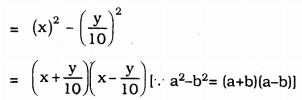9th Maths Polynomials Exercise 4.5 Question 4.
Expand each of the following, using suitable identities :
(i) (x + 2y + 4z)2
(ii) (2x – y + z)2
(iii) (-2x + 3y + 2z)2
(iv) (3a – 7b – c)2
(v) (-2x + 5y – 3z)2
(vi) $$\left(\frac{1}{4} a-\frac{1}{2} b+1\right)^{2}$$
Solution:
(i) (x + 2y + 4z)2
(a + b + c)2 = a2 + b2 + c2 + 2ab + 2bc + 2ca
(x + 2y + 4z)2 =(x)2 + (2y)2 + (4z)2 + 2(x)(2y) + 2(2y)(4z) + 2(4z)(x)
= x2 + 4y2 + 16z2 + 4xy + 16yz + 8zx

(ii) (2x – y + z)2
(x + y + z)2 = x2 + y2 + z2 + 2xy + 2yz + 2zx (2x – y + z)2= (2x)2 + (-y)2 + (z)2 + 2(2x)(-y) + 2(-y)(z) + 2(z)(2x)
= 4x2 + y2 + z2 – 4xy – 2yz + 4zx.

(iii) (-2x + 3y + 2z)2
(x + y + z)2 = x2 + y2 + z2 + 2xy + 2yz + 2zx (-2x + 3y + 2z)2
= (-2x)2 + (3y)22 + (2z)2 + 2(-2x)(3y) + 2(3y)(2z) + 2(2z)(-2x)
= 4x2 + 9x2 + 4z2 – 12xy + 12yz – 8zx

(iv) (3a – 7b – c)2
(a + b + c)2 = a2 + b2 + c2 + 2ab + 2bc + 2ca (3a – 7b – c)2 =(3a)2 + (-7b)2 + (-c)2 + 2(3a)(-7b)
+ 2(-7b)(-c) + 2(-c)(3a) = 9a2 + 49b2 + c2 – 42ab – 14bc – 6ca.

(v) (-2x + 5y – 3z)2
(x + y + z)2 = x2 + y2 + z2 + 2xy + 2yz + 2zx (-2x + 5y – 3z)2 = (-2x)2 + (5y)2 + (-3z)2 + 2(-2x)(5y) + 2(5y)(-3z) + 2(-3z)(-2x) = 4x2 + 25y2 + 9z2 – 20xy – 30yz + 12zx

(vi) $$\left(\frac{1}{4} a-\frac{1}{2} b+1\right)^{2}$$
(a + b + c)2 = a2 + b2 + c2 +2ab + 2bc + 2ca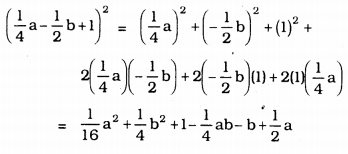Class 9 Maths Chapter 4 Exercise 4.5 Solutions Question 5.
Factorise :
(i) 4x2 + 9y2 + 16z2 + 12xy – 24yz – 16xz
(ii) 2x2 + y2 + 8z2 – 2$$\sqrt{2}$$xy + 4$$\sqrt{2}$$yz- 8xz
Solution:
(i) 4x2 + 9y2 + 16z2+ 12xy – 24yz – 16xz
= (2x)2+ (3y)2 + (-4z)2 + 2(2x)(3y) + 2(3y)(-4z) + 2(-4z)(2x)
= (2x + 3y – 4z)2.

(ii) 2x2 + y2 + 8z2 – 2$$\sqrt{2}$$xy + 4$$\sqrt{2}$$yz- 8xz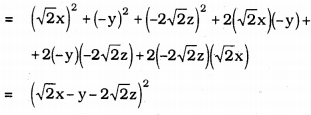KSEEB Solutions For Class 9th Maths Polynomials Question 6.
Write the following cubes in expanded form:
(i) (2x + 1)3
(ii) (2a – 3b)3
(iii) $$\left[\frac{3}{2} x+1\right]^{3}$$
(iv) $$\left[\mathrm{x}-\frac{2}{3} \mathrm{y}\right]^{3}$$
Solution:
(i) (2x + 1)3
As per Identity,
(a + b)3 = a3 + b3 + 3ab(a + b)
(2x + 1)3 = (2x)3 + (1)3 + 3(2x)(1)(2x + 1)
= 8x3 + 1 + 6x(2x + 1)
= 8x3 + 1 + 12x2 + 6x
(2x + 1)3 = 8x3 + 12x2 + 6x + 1.

(ii) (2a – 3b)3
As per Identity,
(a – b)3 = a3 – b3 – 3ab(a – b)
(2a – 3b)3= (2a)3– (3b)3 – 3(2a)(3b)(2a-3b)
= 8a3 – 27b3 – 18ab(2a – 3b)
(2a – 3b)3= 8a3 – 27b3 – 36a2b + 54ab2

(iii) $$\left[\frac{3}{2} x+1\right]^{3}$$
As per Identity,
(a + b)3 = a3 – b3 – 3ab(a + b)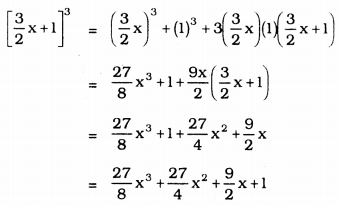(iv) $$\left[\mathrm{x}-\frac{2}{3} \mathrm{y}\right]^{3}$$
As per Identity,
(a – b)3 = a3 – b3 – 3ab(a – b)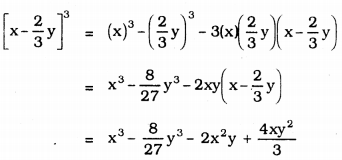KSEEB Solutions For Class 9 Maths Polynomials Exercise 4.5 Question 7.
Evaluate the following using suitable identities :
(i) (99)3
(ii) (102)3
(iii) (998)3
Solution:
(i) (99)3 = (100 – 1)3
As per Identity,
(a – b)3 = a3 – b3 – 3ab(a – b)
(100 – 1)3 = (100)3 – (1)3 – 3(100)(1)(100- 1)
(99)3 = 1000000 – 1 – 300(100 – 1)
= 1000000 – 1 – 30000 + 300
= 970299
∴ (99)3 = 970299

(ii) (102)3 = (100 + 2)3
As per Identity,
(a + b)3 = a3 + b3 + 3ab(a + b)
(100 + 2)3 = (100)3 + (2)3 + 3( 100)(2)( 100 + 2)
= 1000000 + 8 + 600( 100 + 2)
= 1000000 + 8 + 60000 + 1200
(100 + 2)3 = 1061208
∴ (102)3= 1061208

(iii) (998)3 = (1000 – 2)3
As per Identity,
(a – b)3 = a3 – b3 – 3ab(a – b)
(1000 – 2)3 = (1000)3 – (2)3 – 3( 1000) (2)(1000 – 2)
= 1000000000 – 8 – 6000(1000 – 1)
= 1000000000 – 8 – 6000000 + 6000
= 994005992
∴ (998)3 = 994005992

KSEEB Solutions For Class 9 Maths Chapter 4 Question 8.
Factorise each of the following :
(i) 8a3 + b3 + 12a2b + 6ab2
(ii) 8a3 – b3 – 12a2b + 6ab2
(iii) 27- 125a3– 135a + 225a2
(iv) 64a3 – 27b3 – 144a2b + 108ab2
(v) $$27 p^{3}-\frac{1}{216}-\frac{9}{2} p^{2}+\frac{1}{4} p$$
Solution:
(i) 8a3 + b3 + 12a2b + 6ab2
= (2a)3 + (b)3 + 3(2a)(b)(2a + b)
= (2a + b)3 ∵ a3 + b3 + 3ab(a + b) = (a + b)3

(ii) 8a3 – b3 – 12ab2 + 6ab3
= (2a)3 + (-b)3 + 3(2a)(-b)(2a – b)
= (2a – b)3 ∵ a3 – b3 – 3ab(a – b) = (a – b)3

(iii) 27 – 125a3 – 135a + 225a2
OR 125a3 – 225a2 + 135a – 27
= (5a)3 + (-3)3 + 3(5a)(-3)(5a – 3)
= (5a – 3)3 ∵ a3 – b3 – 3ab(a – b) = (a – b)3

(iv) 64a3 – 27b3 – 144a2b + 108ab2
= (4a)3 + (-3b)3 + 3(4a)(-3b)(4a – 3b)
= (4a-3b)3 ∵ a3 – b3 – 3ab(a – b) = (a – b)3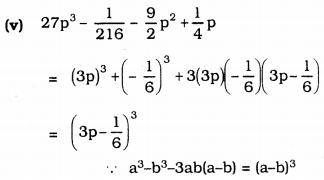Maths Exercise 4.5 Class 9 Question 9.
Verify :
(i) x3 + y3 = (x + y) (x2 – xy + y2)
(ii) x3 – y3 = (x – y) (x2 + xy + y2)
Solution:
(i) x3 + y3 = (x + y)(x2 – xy + y2)
L.H.S. = x3 + y3
R.H.S. = (x + y)(x2 – xy + y2)
= x(x2 – xy + y2) + y(x2 – xy + y2)
= x3 – x2y + xy2 + x2y – xy2 + y3
R.H.S. = x3 + y3
∴ L.H.S. = R.H.S.
∴ x3 + y3 = (x + y)(x2 – xy + y2)

(ii) x3 – y3 = (x – y)(x2 + xy + y2)
L.H.S. = x3 – y3
R.H.S. = (x – y)(x2 + xy + y2)
= x(x2 + xy + y2) – y(x2 + xy + y2)
= x3 + x2y + xy2 – x2y – xy3 – y3
R.H.S. = x3 – y3
∴L.H.S. = R.H.S.
∴ x3 – y3 = (x – y)(x2 + xy + y2)

Polynomials Class 9 KSEEB Solutions Question 10.
Factorise each of the following :
(i) 27y3 + 125z3
(ii) 64m3 – 343n3
[Hint: See Question 9).
Solution:
(i) 27y3 + 125z3
= (3y)3 + (5z)3
= (3y + 5z) {(3y)2 – (3y)(5z) + (5z)2}
= (3y + 5z) (9y2 – 15yz + 25z2)

(ii) 64m3 – 343n3
= (4m)3 – (7n)3
= (4m – 7n) {(4m)2 + (4m) (7n) + (7n)2}
= (4m – 7n) (17m2 + 28mn + 49n2)

Question 11.
Factorise : 27x3 + y3 + z3 – 9xyz
Solution:
27x3 + y3 + z3 – 9xyz
= (3x)3 + (y)33 + (z)3 – 3(3x)(y)(z)
= (3x + y + z) {(3x)2 + (y)2 + (z)2 – (3x)(y) – (y)(z) – (z)(3x)}
= (3x + y + z) (9x2 + y2 + z2 – 3xy – yz – 3zx)

KSEEB Maths Solutions For Class 9 Question 12.
Verify that x3 + y3 + z3 – 3xyz = $$\frac{1}{2}$$ (x + y + z)[(x – y)2 + (y – z)2 + (z – x)2]
Solution:
L.H.S.= x3 + y3+ z3 – 3xyz
R.H.S. = $$\frac{1}{2}$$ (x+y+z) [(x – y)2 + (y – z)2 + (z – x)2]
= $$\frac{1}{2}$$ (x+y+z) [x2 2xy + y2+ y2 – 2yz + z2 + z2 -2zx + x2]
= $$\frac{1}{2}$$ (x+y+z) [2x2+ 2y2+ 2z2– 2xy – 2yz – 2zx]
= $$\frac{1}{2}$$ (x+y+z) × (x2 + y2 + z2 – xy – yz – zx)
= (x + y + z)(x2 + y2 + z2 – xy – yz – zx)
R.H.S.= x3 + y3 + z3 – 3xyz
∴ L.H.S. = R.H.S.
∴ x3 + y3 + z3 – 3xyz = $$\frac{1}{2}$$ (x + y + z)[(x – y)2 + (y – z)2 + (z – x)3]

9th Standard Maths Exercise 4.5 Question 13.
If x + y + z = 0, show that x3 + y3 + z3 = 3xyz.
Solution:
x + y + z = 0
x + y = -z
by cubing on both sides,
(x + y)3 = (-z)3
x3+ y3 + 3xy(x + y) = -z3
x3 + y3 + 3xy (-z) = -z3
(∵ x + y =-z data given)
x3 + y3 – 3xyz = -z3
∴ x3 + y3 + z3 = 3xyz.

KSEEB Solutions For Class 9th Maths Question 14.
Without actually calculating the cubes, find the value of each of the following:
(i) (-12)3 + (7)3 + (5)3
(ii) (28)3 + (-15)3 + (-13)3
Solution:
(i) (-12)3 + (7)3 + (5)3
Let -12 = x, 7 = y, 5 = z, then
x3 + y3 + z3 = 3xyz.
∴ (-12)3 + (7)3 + (5)3
= 3(-12)(7)(5)
= -36 × 35
= -1260.

(ii) (28)3 + (-15)3 + (-13)3
Let x = 28, y = -15, z = -13, then
x3 + y3 + z3 = 3xyz
∴ (28)3 + (-15)3 + (-13)3
= 3(28)(- 15)(-13)
= 84 × 195
= 16380

KSEEB 9th Maths Solutions Question 15.
Give possible expressions for the length and breadth of each of the following rectangles, in which their areas are given :
(i) Area: 25a2 – 35a +12
(ii) Area : 35y2 + 13y – 12
Solution:
(i) Area: 25a2 – 35a + 12
= 25a2 – 20a – 15a + 12
= 5a(5a – 4) – 3(5a – 4)
= (5a -4) (5a – 3)
length × breadth = Area of rectangle
(5a – 4) (5a – 3) = 25a2 – 35a + 12
∴ Length = (5a – 4)
Breadth = (5a – 3)

(ii) Area : 35y2 + 13y – 12
Area of Rectangle – Length × Breadth
35y2 + 13y – 12
35y2 + 28y – 15y
7y(5y + 4) – 3(5y + 4)
(7y – 3) (5y + 4)
∴ Length = (7y – 3)
Breadth = (5y + 4)

KSEEB Solutions Class 9 Maths Question 16.
What are the possible expressions for the dimensions of the cuboids whose volumes are given below :
(i) Volume: 3x2 – 12x
(ii) Volume: 12ky2 + 8ky – 20k
Solution:
(i) length × breadth × height = Volume of Cuboid
= 3x2 – 12x
= 3x2 – 12x
= 3x(x – 4)
= 3 × x × (x – 4)
l × b × h
∴ Length = 3
Height = (x – 4)

(ii) length × breadth × height = Volume of Cuboid
= 12ky2 + 8ky – 20k
12ky2 + 8ky – 20k
= 4k(3y2 + 2y – 5)
= 4k (3y2 + 5y – 3y – 5)
= 4k (y(3y + 5) – 1 (3y + 5))
= 4k × (3y + 5) × (y – 1)
= l × b × h
∴ Length = 4k
Breadth = (3y + 5)
Height = (y – 1)

We hope the KSEEB Solutions for Class 9 Maths Chapter 4 Polynomials Ex 4.5 help you. If you have any query regarding Karnataka Board Class 9 Maths Chapter 4 Polynomials Exercise 4.5, drop a comment below and we will get back to you at the earliest.